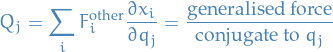# Lagrangian Dynamics

## Notation

•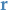position vector of particle of mass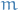•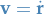is velocity
•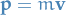is linear momentum
•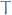is kinetic energy
•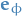is the unit vector perpendicular to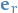(the unit position vector)

## Equations / Theorems

### Galilean Transformation

Transforms from one inertial frame to another moving at constant velocity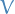relative to it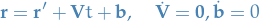Then,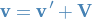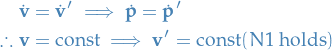### Conservation laws

#### Angular momentum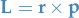##### Proof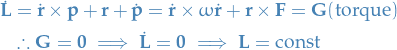Remember, in this case we have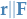and thus $×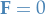### Lagrange's equations Equations of motion in terms of generalised coordinates.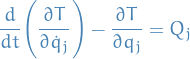• equivalent to Newton's laws • constraint equation have been eliminated • constraint forces do not appear - they don't contribute to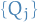Remember that the generalized forcesare simply the forces projected onto the generalized coordinates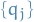. #### Derivation We restrict our attention to functions of the form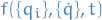, where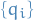denotes the generalised coordinates. We assume Newton's Laws to be true in this derivation. Since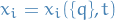we can apply the "cancellation of dots" which just says that for the case where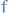is not a function of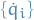, then we have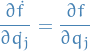thus,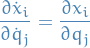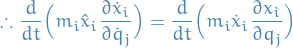Further,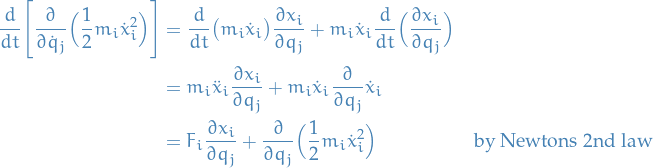Summing over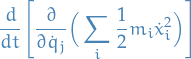we see that it's just the kinetic energyas a function of the generalised coordinates, velocities and time. Thus,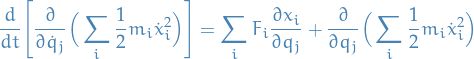where the first term is just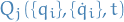. Since the constraint forces do now work, the sum over all constraint forces is zero. Therefore we end up with Lagrange Equations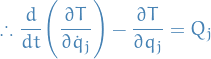#### Using conservative forces If the forces are conservative there exists a potential energy function such that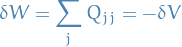Assume$V = V(\{qj\}, t) then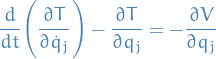Since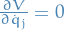, we may write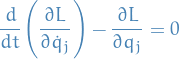where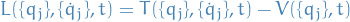This is for holonomic constraints.

This applies to systems which also does not conserve their energy, unlike the usual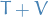which is only valid for systems which conserve energy.

##### Examples
• Single particle in Cartesian Coordinate

We have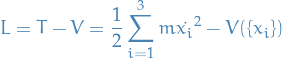Substituting into Lagrange's equation for conservative forces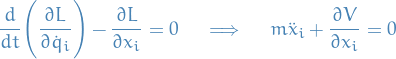• Single particle in polar coordinates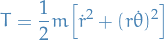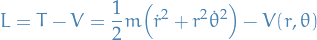Substituing into Lagrange's equations wrt.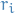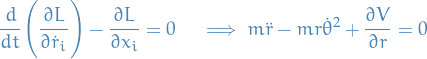and wrt.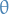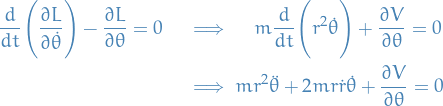## Definitions

### Intertial frame

A frame in which Newtons 1st and 2nd laws hold.

### Newtons Laws

#### Newtons 3rd law

##### Weak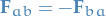for two objects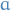and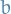acting on each other.

##### Strong

Weak assumption AND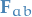acts along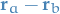### Effect potential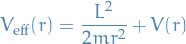due towhere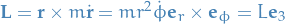gives us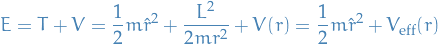### Constraint force

Constraint forces do no work in any small instantaneous displacement of the system consistent with the constraints themselves.

Does not mea that the constraint forces can do no work during the actual motion of the system, e.g. a particle constrained to lie on a surface which is itself moving: there may then be a component of the actual particle velocity in the direction of the constraint force, so that work is done.

### Circular orbits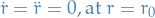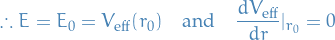### Holonomic constraints

#### Example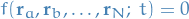i.e. it's a algebraic equation between the coordinates, not a differentiable relation and not an inequality.

### Generalised coordinates

Consider a system with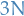coordinates, i.e. 3D with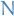particles each with coordinates, and let these coordinates be denoted by $xi,$ where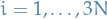.

That is, we're just "flattening" the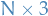matrix to avector.

If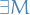holonomic constraints, not all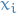are independent, and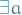set of independent variables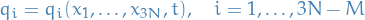We might have dependence between thedue to the constraints being imposed, and thus representing the coordinates in the above way is just "removing" the dependence between the.

Hence, we end up with a basis of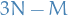dimensions.

Our aim is to derive2nd order differential eq. for the set of generalised coordinates.

Transformation from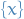tois invertible using constraint eq., that is: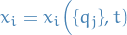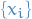cannot be varied independently without violating the constraints, whereas we can varywhile still satisfying the constraints.

#### Generalised velocities

Ifdenotes the generalised coordinates, thenrepresents the generalised velocities.

#### Generalised forces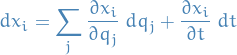Here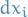may have a component in the direction of a constraint force, due to motion, and so the constraint force may do work, e.q. a body on a surface is utself moving.

"Instantaneous", i.e.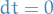Then a small change consistent with constraints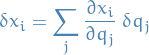In a virtual displacement the work done is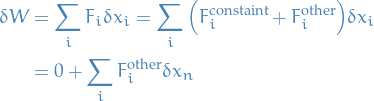since constraint forces do no work.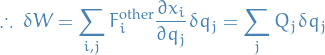where